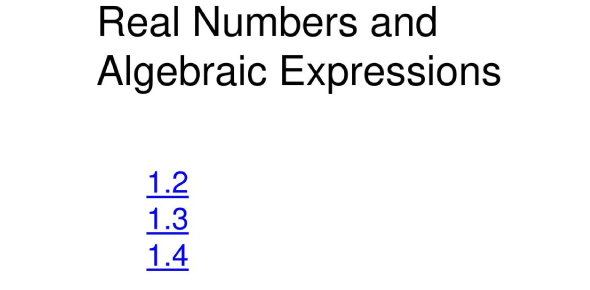# Real Numbers And Algebraic Expressions Quiz! Trivia

10 Questions | Total Attempts: 176Settings.

Related Topics
• 1.
The complex number system is divided into two groups of numbers - real and imaginary.
• A.

True

• B.

False

• 2.
A rational number can't be a decimal that repeats.
• A.

True

• B.

False

• 3.
All square roots are irrational numbers.
• A.

True

• B.

False

• 4.
Which is the algebraic expression for twelve less than x?
• A.

X - 12

• B.

12 - x

• 5.
What is the algebraic expression for the product of 6 and t?
• A.

6t

• B.

6/t

• C.

6 - t

• D.

T - 6

• 6.
Which statement below describes this equation? 5(x - 4)
• A.

Five times x minus 4

• B.

The product of five and the difference of x and 4

• C.

Five x less than 4

• 7.
The set of positive and negative numbers are called
• 8.
Decimals that do not stop are called ________________________
• 9.
8 / 24 is a reduced fraction.
• A.

True

• B.

False

• 10.
Rational numbers are the numbers that can be written as a _______________